Study Guide

# In the Real World

## In the Real World

We've seen that geometric series can get used to calculate how much money you've got in the bank. They can also be used to calculate the amount of medicine in a person's body, if you know the dosing schedule and amount and how quickly the drug decays in the body.

On a more fun note, the harmonic series is a divergent infinite series. There's also a harmonic series in music, and they're very closely related.

When you pluck a string on a musical instrument, it creates more than one note. It creates a base note and also a collection of higher notes called harmonics or the (musical) harmonic series.

The different musical harmonics correspond to the different terms of the mathematical harmonic series.

When a string is plucked, it vibrates along its whole length to form the base note.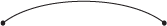At the same time, it vibrates in two pieces to form the first harmonic of the musical harmonic series.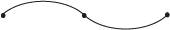It vibrates in three pieces to form the next harmonic of the musical harmonic series.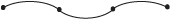And so on, and so on.

If we say the original length of the string is 1, then the base note and harmonics of the musical harmonic series correspond to the lengths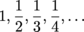These are the terms of the mathematical harmonic series.

This video demonstrate the musical harmonics inside a piano.

This page has an interactive demo that lets you see how the string vibrates and hear the corresponding musical harmonics at the same time.

• ### I Like Abstract Stuff; Why Should I Care?

You've known for a long time that addition is commutative. No matter what order you add the numbers, you'll always get the same answer. Well... that's true when you're adding finitely many numbers.

When you're adding infinitely many numbers, the rules change. If a series converges absolutely you can still rearrange the terms however you want. But if a series converges conditionally, rearranging the terms can make them add up to different values. In fact, the terms can be rearranged to add up to anything you want.

The alternating harmonic series is a good example of this weirdness. The alternating harmonic series is conditionally convergent, and when we get to Taylor series we'll see that it sums to ln 2. In symbols,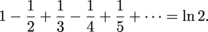Now let's rearrange the terms. We can write the terms of the alternating harmonic series like this: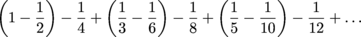First take a moment to convince yourself that all the terms of the alternating harmonic series do show up in this rearrangement.

Then simplify the stuff in parentheses to get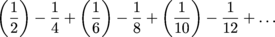Factor out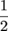from each term (that is, factor out 2 from each denominator). Now we have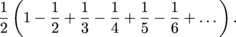This looks familiar. It'smultiplied by the alternating harmonic series. Since the sum of the alternating harmonic series is ln 2,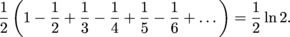By rearranging the terms, we made them add up to only half of what they added up to before.

That was only one example. What if we wanted to make the terms add up to 7? Or -32? This article gives a nice explanation of why we can make the terms add up to anything we want:

• ### How to Solve a Math Problem

There are three steps to solving a math problem.

1. Figure out what the problem is asking.

2. Solve the problem.

### Sample Problem

The Cantor set, denoted c, is a particular subset of the interval [0,1].

Our favorite way to describe c is as the limit of a particular sequence of sets C0, C1, C2, C3, ....

Let C0 be the unit interval [0,1]: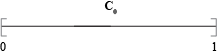To get C1, remove the open interval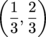from the middle of C0: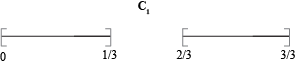The set C1 consists of two line segments, each of length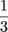.

To get C2, remove the open interval that forms the middle third of each of those line segments: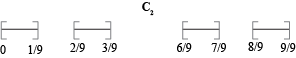The set C2 consists of four line segments, each of length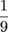.

We keep going this way for all eternity, and define the Cantor set by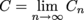This means c consists of the points that are in all the sets Cn.

Let the set C be the complement of the Cantor set - that is, all points in [0,1] that aren't in c.

Find the length of C. What does this tell us about the length of c?

1. Figure out what the problem is asking.

There are a lot of words here, but what's really going on?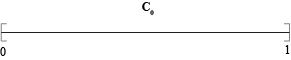We're going to take out a bunch of stuff and throw everything we take out into the set C.

Every time we remove the middle of a line segment, the portion we remove goes into C: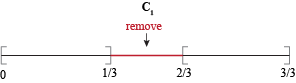The question is asking us to find the length of C. In other words, it's asking for the sum of the lengths of all the line segments we remove along the way.

2. Solve the problem.

We need to add up the lengths of all the line segments we remove. On the first step, we remove one line segment of length.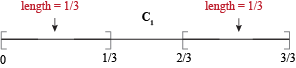On the second step we remove two line segments, each of length. The sum of their lengths is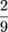.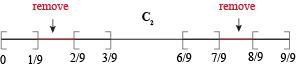On the third step we remove four line segments, each of length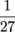. The sum of their lengths is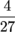.

At this point we can see the pattern:The total length of the line segments we remove from step 1 to step n is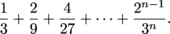In the limit, the total length of all the line segments we remove is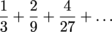This is an infinite geometric series with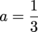and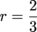, so its sum is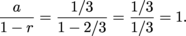The total length of all the line segments we remove is 1. This means the length of C is 1.

Since C is everything that's not in c, this suggests that the length of c is 0.

We don't have a great way to check the answer to this one, but we can at least think about whether it makes sense.

We found that the length of c is 0. This means c can't contain any line segments at all.

Well, c certainly doesn't contain any line segments longer thanbecause after step 1 the longest line segments remaining have length. c also doesn't contain any line segments longer thanbecause after step 2 the longest remaining line segments have length.

Continuing like this we realize c can't contain any line segments longer than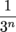, for any value of n.

This means that c can't contain line segments of any length greater than 0. Consider any non-zero length L. There's a value of the formthat's smaller than L, which means L is too big for c to contain any line segments of that length.

Since no line segments of any non-zero length exist in c, it makes sense to say that "the length of c is 0."

Although the length of the Cantor set c is 0, it does contain points. The Cantor set contains, at the very least, the points 0, 1,, and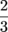, so we can see that it's not empty. In fact, it turns out that the Cantor set contains uncountably many points.

The Cantor set has length 0, but uncountably many points. Does your brain hurt yet?